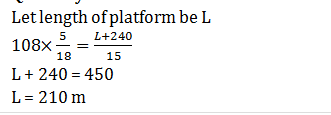# Train related Quant Quiz for SBI PO PRE 2019 (Day 20) | 23rd February 2019

## Train related Quant Quiz for SBI PO PRE 2019 (Day 20)

Quant Quiz to improve your Quantitative Aptitude for SBI PO & SBI clerk exam quant, IBPS PO quant , IBPS Clerk quant , IBPS RRB quant, and other competitive exams.

Q1. A 240 m long train takes 40 s longer to cross a platform twice its length than the time it takes to cross a pole at the same speed. What is the speed of the train?

A) 6 m/s

B) 24 m/s

C) 48 m/s

D) 12 m/s

E) None of these

Q2.  The distance between two stations A and B is 900 km. A train starts from A and moves towards B at an average speed of 30 km/hr. Another train starts from B, 20 minutes earlier than the train at A, and moves towards A at an average speed of 40 km/hr. How far from A will the two trains meet ?

A) 380 km

B) 320 km

C) 240 km

D) 330 km

E) None of these

Q3. Two trains were running in opposite directions at the speed of 48 kmph and 24 kmph respectively. If the faster train passed a man sitting in the slower train in 9.5 seconds, what is the length of the faster train?

A) 180m

B) 190m

C)  210m

D) 240m

E)  None of these

Q4. The length of train A is twice that of train B and speed of train A is half of that of train B. If train A crosses a man in 4 sec. then find how long will train B take to cross train A if they go in same direction.

A) 3s

B)  4s

C) 5s

D) 6s

E) None of these

Q5. Trains A and B are travelling at x km/hr and (x + 36) km/hr respectively. Train B crosses train A when running in the same direction inseconds. Find the sum of speed of both the trains if they pass each other in 9 seconds while running in opposite direction

A) 126 km/hr

B) 120 km/hr

C) 116 km/hr

D) 136 km

E)  115 km/hr

Q6. A train running at the speed of 108 km/hr crosses a pole in 8 sec. Find Length of train & Length of platform which train crosses in 15 sec.

A) 180 m & 200 m

B) 200 m & 150 m

C) 220 m & 160 m

D) 240 m & 180 m

E) 240 m & 210 m

Q7. The ratio between length of two goods train is 5 : 4. If both trains running in same direction at the speed of 144 km/hr and 108 km/hr and faster train cross the slower train in 54 sec, then find in what time both goods train cross each other, when running in opposite direction?

A) 54/7 sec

B) 44/7 sec

C) 58/7 sec

D) 62/7 sec

E) 60/7 sec

Q8. Train A running at the speed of 108 km/hr crosses a man, who running in the opposite direction at the speed of 12 km/hr in 7.2 sec. If speed of train A increased by 25% and it takes 48 seconds to cross another train B, which running at the speed of 90 km/hr in same direction. Find the length of train B?

A)  280 meters

B) 360 meters

C) 180 meters

D) 160 meters

E) 220 meters

Q9.  A 240 meters long train crosses a 210 meters long train running in opposite direction in 6 sec. Ratio between speed of longer train and smaller train is 7 : 8. If faster train crosses a platform in 9 sec, then find time taken by slower train to cross a bridge, which is 60 meters more long than platform?

A) 142/7 sec

B) 136/7 sec

C) 90/7 sec

D) 148/7 sec

E) 141/7 sec

Q10. Two trains start at the same time from Allahabad and Kanpur and proceed towards each other at the rate 73 km and 47 km per hour respectively. When they meet, it is found that one train has travelled 13 km more than the other. Find the distance between Kanpur and Allahabad.

A) 70 km

B) 60 km

C) 75 km

D) 65 km

E) 80 km

## Solutions

Q1. Ans(D)Q2. Ans(A)Q3. Ans(B)Q4. Ans(D)Q5. Ans(A)Q6. Ans(E)Q7. Ans(A)Q8. Ans(B)Q9. Ans(C)Q10. Ans(B)## WhatsApp Group Join here

Mail us at : ambitiousbaba1@gmail.com